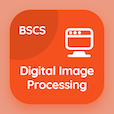SAT Online Courses

SAT Physics MCQs

SAT Physics MCQ PDF - Topics

# Speed Velocity and Acceleration MCQ Quiz Online

Learn Speed Velocity and Acceleration Multiple Choice Questions (MCQ), Speed Velocity and Acceleration quiz answers PDF to study sat physics online course for sat physics classes. Kinematics Multiple Choice Questions and Answers (MCQs), Speed Velocity and Acceleration quiz questions for free career test. "Speed Velocity and Acceleration MCQ" PDF Book: speed velocity and acceleration, speed time graphs, distance time and speed test prep for free career quiz.

"A football rolling in a straight line slows down from 10 ms-1 to 4 ms-1 in 15 s. Its acceleration is" MCQ PDF: speed velocity and acceleration with choices 0.4ms-2, −0.4 ms-2, 2 ms-2, and 20 ms-2 for free career test. Study speed velocity and acceleration quiz questions for merit scholarship test and certificate programs for questions to ask during an interview.

## MCQs on Speed Velocity and Acceleration Quiz

MCQ: A football rolling in a straight line slows down from 10 ms-1 to 4 ms-1 in 15 s. Its acceleration is

0.4ms-2
−0.4 ms-2
2 ms-2
20 ms-2

MCQ: The SI unit of speed is

mh-1
ms-1
ms-2
kmh-1

MCQ: The change in velocity in the opposite direction as the velocity is called

constant acceleration
zero acceleration
negative acceleration
positive acceleration

MCQ: The change in velocity in the same direction as the velocity is called

constant acceleration
zero acceleration
negative acceleration
positive acceleration

MCQ: The change in velocity with the time is termed as

distance
acceleration
speed
displacement

### More Topics from SAT Physics Course